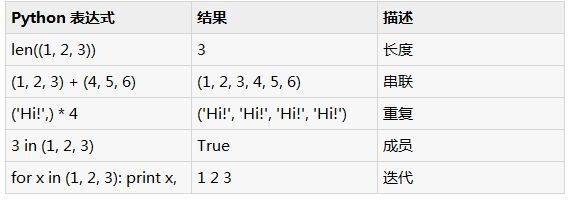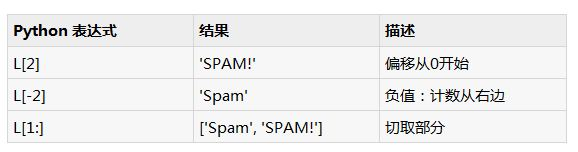# 初步?#27493;釶ython中的元组概念

```tup1 = ('physics', 'chemistry', 1997, 2000);
tup2 = (1, 2, 3, 4, 5 );
tup3 = "a", "b", "c", "d";

```

```tup1 = ();

```

```tup1 = (50,);

```

```#!/usr/bin/python

tup1 = ('physics', 'chemistry', 1997, 2000);
tup2 = (1, 2, 3, 4, 5, 6, 7 );

print "tup1: ", tup1
print "tup2[1:5]: ", tup2[1:5]

```

```tup1: physics
tup2[1:5]: [2, 3, 4, 5]

```

```#!/usr/bin/python

tup1 = (12, 34.56);
tup2 = ('abc', 'xyz');

# Following action is not valid for tuples
# tup1 = 100;

# So let's create a new tuple as follows
tup3 = tup1 + tup2;
print tup3;

```

```(12, 34.56, 'abc', 'xyz')

```

?#22659;?#30340;元组元素：

```#!/usr/bin/python

tup = ('physics', 'chemistry', 1997, 2000, hema);

print tup;
del tup;
print "After deleting tup : "
print tup;

```

```('physics', 'chemistry', 1997, 2000)
After deleting tup :
Traceback (most recent call last):
File "test.py", line 9, in
print tup;
NameError: name 'tup' is not defined

``````L = ('spam', 'Spam', 'SPAM!')
``````#!/usr/bin/python

print 'abc', -4.24e93, 18+6.6j, 'xyz';
x, y = 1, 2;
print "Value of x , y : ", x,y;

```

```abc -4.24e+93 (18+6.6j) xyz
Value of x , y : 1 2

```

Tools Online | 在线开发工具
RankList | 热门文章Recommend | 推荐阅读
PHP1.CN | 中国最专业的PHP中文社区 | PHP资讯 | PHP教程 | 数据库技术 | 服务器技术 | 前端开发技术 | PHP框架 | 开发工具 | PHP问答